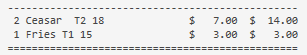# Show Taxes per order line

Is it possible to print the tax amount per order line?

{ORDER TAX RATE:X} could possible be used in a calculation if I did not have to specify X

If you didnt specify the tax type how would it know which tax to use?

Sure just use an expression for it.

Because it is assigned through mappings in the tax template.

For example if I use `{ORDER TAX RATE:23% Vat}` it returns 23 (I could just use 23, I don’t see the benefit of this print tag) but some items have 13.5% Vat applied so it is of no use.

[quote=“kendash, post:3, topic:15383, full:true”]
Sure just use an expression for it.
[/quote] How can I pull the orders tax rate to use it in an expression.

Also on some ticket types taxes may not be applied at all so it should return 0

I need something like `{ORDER TAX RATE}` without the X

By expression I mean manually calculate it using math.

Or use parameters in the tax tag and use a list of tax templates.

Like this (my Template Names are “T1” and “T2” which are 15% and 18%, respectively):

``````[ORDERS]
<W00: 2,1,*, 3, 7, 3, 7>{QUANTITY}||{NAME} [T1 {ORDER TAX RATE:T1}] [T2 {ORDER TAX RATE:T2}]|\$|{PRICE}|\$|{TOTAL PRICE}
{ORDER TAGS}
``````

The outer `[square brackets]` makes it conditional on whether a value exists. If no value exists, nothing is printed, so this part …

``````[T1 {ORDER TAX RATE:T1}] [T2 {ORDER TAX RATE:T2}]
``````

Will print the rate for T1 if it exists, or nothing, and it will print the rate for T2 if it exists, or nothing.

The output in this case looks like the following, where “Ceasar” is a T2 Product, and Fries is a T1 Product …1 Like